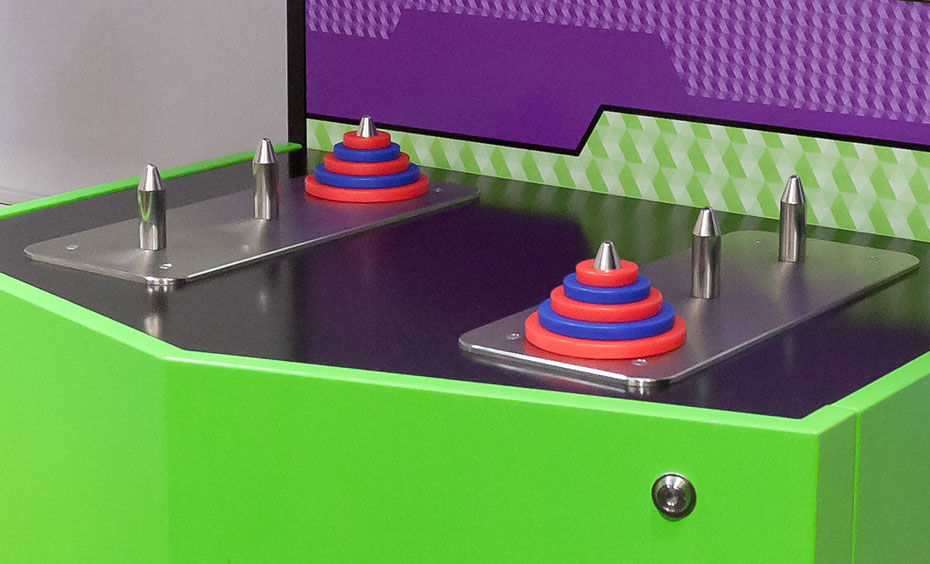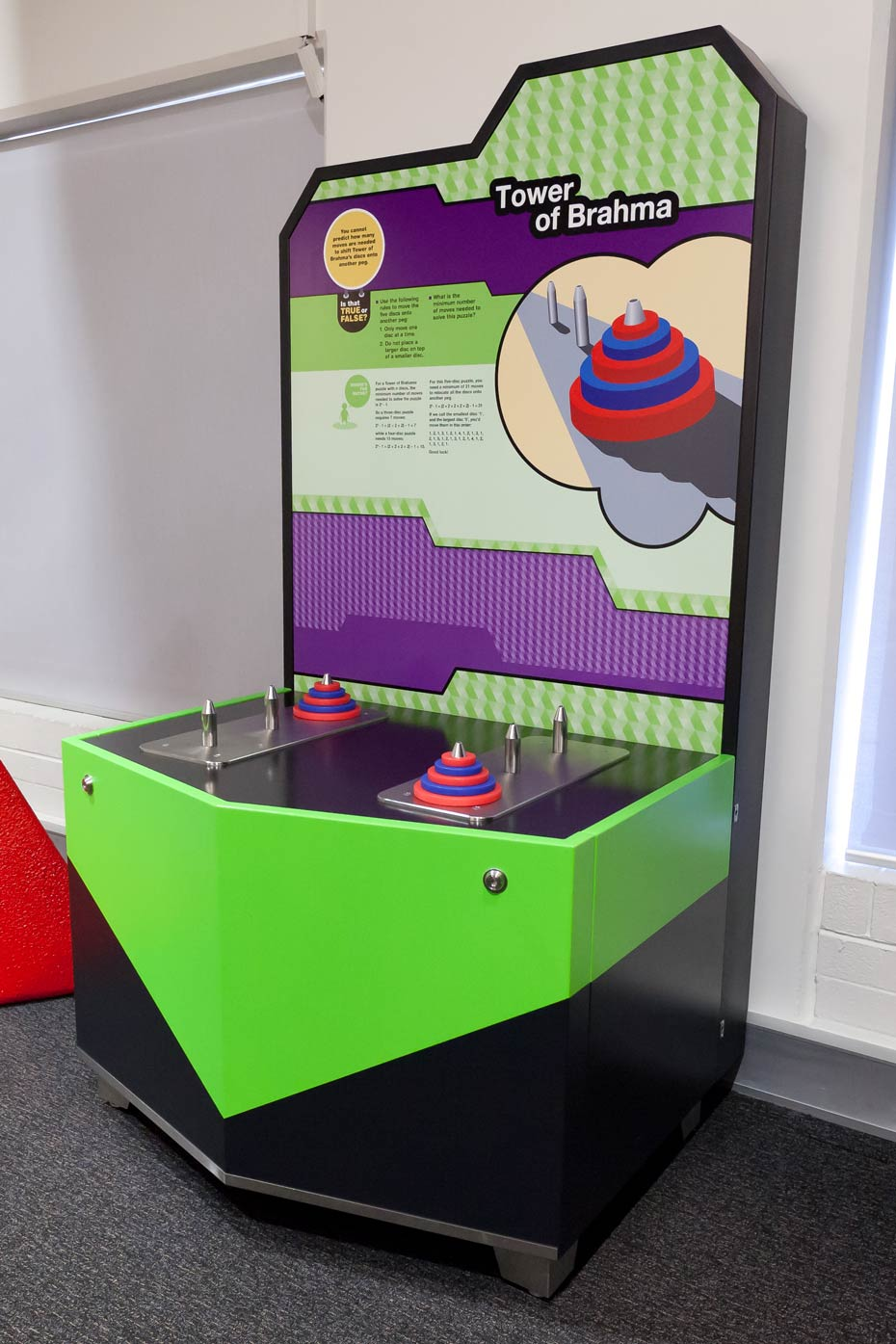# Tower of BrahmaThe minimum number of moves required to solve a Tower of Brahma puzzle depends on the number of discs being used.

## How it works

You must relocate a stack of five discs onto another peg, following two rules: move one disc at a time and do not place a larger disc on top of a smaller disc. Can you solve the puzzle in a minimum number of moves?

## Things to try or ask around the exhibit

• Can we solve the puzzle in a minimum number of moves?
• Let's try the puzzle with three discs. How can the number of discs be used to calculate the minimum number of moves?

## Background

For a Tower of Brahma puzzle with n discs, the minimum number of moves needed to solve the puzzle is 2n - 1. For a four-disc Tower of Brahma puzzle, you need a minimum of 15 moves to relocate all the discs onto another peg.

A three-disc puzzle requires a minimum of 7 moves: 23 - 1 = (2 × 2 × 2) - 1 = 7 while a five-disc puzzle needs a minimum of 31 moves: 25 - 1 = (2 × 2 × 2 × 2 x 2) - 1 = 31.